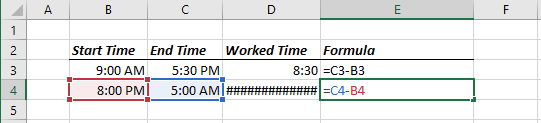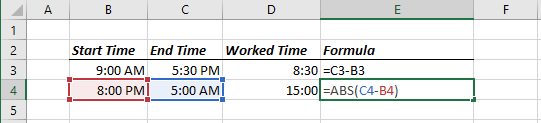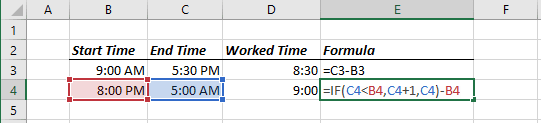# Calculating the difference between two time stamps

Excel
Because timestamps are represented as serial numbers, you can subtract the earlier time from the later time to get the difference.

For example, if cell B3 contains 9:00:00 and cell C3 contains 17:30:00, the following formula returns 08:30:00 (a difference of eight hours and 30 minutes):

= C3 - B3If the subtraction results in a negative value, however, it becomes a wrong time; Excel displays a series of hash marks (#######) because time without a date has a date series number of 0. A negative time results in a negative series number, which is not permitted.

If the direction of the time difference doesn't matter, you can use the ABS function to return the absolute value of the difference:

= ABS (C4 - B4)This negative time problem often occurs when calculating an elapsed time - for example, calculating the number of hours worked given a start time and an end time. This presents no problem if the two times fall on the same day. But if the work shift spans midnight, the result is a negative time.

For example, you may start work at 08:00 PM, and end work at 5:00 AM the next day. Using the ABS function (to calculate the absolute value) isn't an option in this case because it returns the wrong result (15 hours). The following formula, however, does work:

= IF (C4 < B4, C4 + 1, C4) - B4In fact, another formula (even simple) can do the job:

= MOD (C4 - B4, 1)

See also this tip in French: Calculer la différence entre deux temps.

If you have any questions or suggestions, please feel free to ask OfficeToolTips team.